# Complex Numbers for Programmers

Okay. Let’s talk about something that any CS grad will know but not necessarily like or remember. Many will hate it and forget about it as soon as they can and most will never work with it in their life. A topic that makes H. P. Lovecraft’s Cthulu shudder because it’s so messed up.
We will be talking about , no not the language, that doesn’t have a funky additional vertical line, but about Complex Numbers. And since we are programmers, not mathematicians, the whole thing has suddenly become way less intimidating! (Albeit not less irrational, mind you.)

## Introduction to complex numbers

A Complex Number is a weird thing. First off, it’s not on any one-dimensional number line. Meaning, we can’t count Complex Numbers like normal numbers. (1…2… Freddy’s Coming For You).
Instead, Complex Numbers are composed of two main components, namely the real part ( or  and the imaginary part ( or  b, which is always multiplied by i. The weird thing about this is, that i, the so called complex unit, satisfies i2 = -1.

You may now call bullshit. But this assumption is actually really important in many areas. (Read: Chaos Theory, Relativity, Quantum Mechanics, Signal Processing… etc.)

For example, i2 = -1 allows us to solve negative square roots. The square root of -25 is nothing else but 5i, which is a pure imaginary number. However, it is also a complex number.

The notation for complex numbers is usually: a + bi where a is the real part and b the imaginary part.

The square root of -25 in this notation is thus. 0 + 5i.

So when in highshool your result was a negative square root, you could have continued to do the maths for that problem. Most of the time it wouldn’t make your result any more correct, but you could have if you wanted to.

## The Complex Plane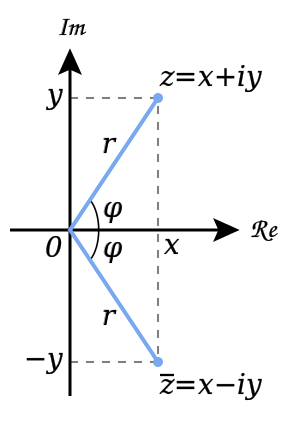So I mentioned that Complex Numbers are not one-dimenensional. Since they have two components it is a safe guess to assume that they are infact two-dimensional (or can be interpreted as such). Each Complex Number lies on the so called Complex Plane. (Image with courtesy to Wikipedia).
Using the real part of a Complex Number as the x value and the Imaginary part as the y value we can map each complex number to a spot on a two-dimensional plane.
I don’t know about you, but this looks like vectors to me.

And as programmers we do know our vector math!

However, there is another way of describing a Complex Number than the a + bi style, which is also known as the Coordinate Form. Since we established a mapping of any Complex Number zto a point p on a plane we can describe our complex number as an angle to the x axis and the distance of our point from the origin of our plane.

Now, we can further abbreviate this using the Euler Formula. Euler was a smart man you see, he proclaimed that  which allows us to use the magical concept of substitution and insert  for  which in turn shortens the whole equation to: .

Amazing man, that Euler guy.

This is also called the Polar Form of a Complex Number.

Furthermore, we can convert our standard notation to Polar Form quite easily by calculating the distance to 0 and the angle.

Good ol‘ lad Pythagoras will help with the distance. We are after all looking at a 2D plane, which means that any distance from the origin can be calculated by a right-angled triangle.

This is also called the Modulus of a Complex Number.

### Coordinate Form to Polar Form

Given Normal (Coordinate) Form:

Calculate r (distance from zero)

Calculate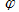(Angle from the positive x axis) (which is about 59.04 degrees)

Polar Form

Oh look! Something that looks like an euler function! We can of course reverse this process…

### Polar Form to Coordinate Form

Polar Form

Calculate the Real Component of z

Calculate the Imaginary Component of z

Coordinate Form

You may have noticed a new notation here.  is commonly understood as „The real part of z“ (the a in z = a + bi), whereas  can be read as „The imaginary part of z“, (the b in z = a + bi).

Keep that in mind, you’ll need it in a second.

## Addition, Subtraction, Multiplication, Division of complex numbers…

Being able to write and convert Complex Numbers is nice and all, but most often you will have to use them in computations. So how on earth do we do the maths with complex numbers? Well, if you ever worked with vectors this will be awfully familiar. If not, I am sure Olaf is burning to see you covered with a „Vector Math and Trig for Programmers“ post.

We will only cover calculation in Coordinate Form here, we don’t really need the other cases if we can simply convert everything in no time on a cpu. We are, after all, lazy programmers. (Don’t lynch me please.)

Addition of the complex numbers  and  to a new Complex Number  is defined as follows:

Analogous, subtraction is defined as

In short, addition of complex numbers in 2d coordinate form is exactly the same thing as adding a two-dimensional vector to another. Multiplication of said numbers is a bit different, but it’s no less similar to something we already know very well.

Multiplication of the complex numbers  and  to a new Complex Number  is defined as follows:

As we can see, the real part is nothing but a normal multiplication of the respective real and imaginary parts, then subtracting the result. The imaginary part of z is something akin to a cross product! And lastly:

Analogous, Division of the complex numbers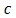and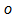to a new Complex Number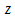is defined as follows:

The numerator of each division is basically the same thing as the multiplication, but we subtract instead of adding and vice versa. The denominator is new, looks very pythagorian (if you ask me) and I am sure that you can find mathemathical proof on the net for this if you are interested. As for now, there is no need to prove anything to you guys! Take my word or leave! (Please stay, I need your connection time for SEO!)

## Code!

I called this „Complex Numbers for Programmers“, so naturally you are going to get some code at the end. The code itself is very self explanatory, have fun using it, but remember, using wrapped classes for calculations comes at a performance cost.

Thanks for your time and see you in the next post!

public struct ComplexNumber {
/// <summary>
/// Real Part of the Complex Number
/// </summary>
public double Real;

/// <summary>
/// Imaginary Part of the Complex Number
/// </summary>
public double Imaginary;

/// <summary>
/// Phi of the Complex Number used in Planar Form
/// </summary>
public double Phi {
get {
return Math.Atan2(Imaginary, Real);
}
}

/// <summary>
/// Returns the Conjugate Complex number
/// </summary>
public ComplexNumber Conjugate {
get {
return new ComplexNumber(Real, -Imaginary);
}
}

/// <summary>
/// Creates Complex Number object
/// </summary>
/// <param name="real"></param>
/// <param name="imaginary"></param>
public ComplexNumber(double real, double imaginary) {
Real = real;
Imaginary = imaginary;
}

/// <summary>
/// Create a Complex Number object from it's Polar Form
/// </summary>
/// <param name="r"></param>
/// <param name="phi"></param>
/// <returns></returns>
public static ComplexNumber FromPolar(double r, double phi) {
return new ComplexNumber(
r * Math.Cos(phi),
r * Math.Sin(phi)
);
}

/// <summary>
/// Returns the distance of this Complex Number from the origin of the Complex plane
/// </summary>
public double Modulus {
get {
return Math.Sqrt((Real * Real) + (Imaginary * Imaginary));
}
}

/// <summary>
/// Returns the squared distance of this Complex Number from the origin of the Complex Plane
/// </summary>
public double SqrModulus {
get {
return (Real * Real) + (Imaginary * Imaginary);
}
}

public static ComplexNumber operator +(ComplexNumber a, ComplexNumber b) {
return new ComplexNumber(a.Real + b.Real, a.Imaginary + b.Imaginary);
}
public static ComplexNumber operator -(ComplexNumber a, ComplexNumber b) {
return new ComplexNumber(a.Real - b.Real, a.Imaginary - b.Imaginary);
}
public static ComplexNumber operator *(ComplexNumber a, ComplexNumber b) {
return new ComplexNumber((a.Real * b.Real) - (a.Imaginary * b.Imaginary),
(a.Imaginary * b.Real) + (a.Real * b.Imaginary));
}
public static ComplexNumber operator /(ComplexNumber a, ComplexNumber b) {
return new ComplexNumber(((a.Real      * b.Real) + (a.Imaginary * b.Imaginary)) / ((b.Real * b.Real) + (b.Imaginary * b.Imaginary)),
((a.Imaginary * b.Real) - (a.Real      * b.Imaginary)) / ((b.Real * b.Real) + (b.Imaginary * b.Imaginary)));
}

public static bool operator ==(ComplexNumber a, ComplexNumber b) {
return (a.Real == b.Real && a.Imaginary == b.Imaginary);
}

public static bool operator !=(ComplexNumber a, ComplexNumber b) {
return !(a == b);
}

public override bool Equals(object obj) {
if (obj is ComplexNumber) {
return this == (ComplexNumber)obj;
}
return false;
}

public override int GetHashCode() {
return base.GetHashCode();
}

#endregion

}